# There 12

There are 42 students in the class and 2/3 of them are girls. How may girls are there in the class?

g =  28

### Step-by-step explanation:Did you find an error or inaccuracy? Feel free to write us. Thank you!Tips to related online calculators
Need help to calculate sum, simplify or multiply fractions? Try our fraction calculator.

## Related math problems and questions:

• FortyForty five of the 80 students were girls. What is the ratio of girls to boys?
• Pupils 7There are 40 pupils in a certain class. 3/5 of the class are boys. How many are girls?
• One third 2One third of all students in class live in a house. If here are 42 students in a class, how many of them live in house?
• StudentsThere are 2/6 girls out of 1050 students at the school. Of these, 4/5 have another sibling. How many girls are in school? How many boys are in school? How many girls do not have a sibling?
• English and math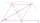1/5 of a class study English, and 3/5 study mathematics. If the class consist of 80 students, how many students study English and mathematics in the class?
• The ratioThe ratio of girls to boys in Mrs. White's class is 3:2.  If there are 12 boys, how many girls are in the classroom?
• Math test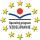In class 6.A are 26 students, and the teacher had managed to give tests to 13 students. 13 testes he gave in 6.5 min. How many pupils still haven't test? How long will it take the teacher to give tests to 43 students?
• Prospering studentsThere are girls and 30 boys in the class. Boys prospering 28, girls all. How many girls are in the class and how many boys are there, if all the prospering students are 95%?
• ShirtsIn a classroom, 1/6 of the students are wearing blue shirts and 2/3 are wearing white shirts. There are 36 students in the class. How many students are wearing a shirt other than blue or white?
• Class 8.AThree quarters of class 8.A went skiing. Of those who remained at home one third was ill and the remaining six were on math olympic. How many students have class 8.A?
• Classroom 4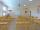In a class of 36 pupils, 2/3 are girls. How many girls and boys are in the class?
• Students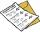The class has 22 students. 2 of which have assessments of 2. The count of assessment of 1 is three times more than the count of students with an assessment of 3. How many students have an assessment of 1, and how many students have an assessment of 1.
• Boys and girlsIf the ratio of male & female teachers is 2/5 & it is in proportion of the male & female students, how many are girls if there are 42 boys?
• Report 2A School reports students to teacher ratio of 6:1. If there are 45 teachers in the School, how many students are there?
• Class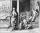When Pythagoras asked how many students attend the school, he said: "Half of the students studying mathematics, 1/4 music, seventh silent, and there are three girls at school." How many students had Pythagoras at school?
• A class IV.AIn a class there are 15 girls and 30 boys.  What fraction of the class represents the boys?
• GroupsIn the 6th class there are 60 girls and 72 boys. We want to divide them into groups so that the number of girls and boys is the same. How many groups can you create? How many girls will be in the group?